JAVA, HTML5 & Chemistry Site

Other Writing

Last Update
19-Oct-2014

### Properties Estimation: Estimation of Refractive Index part 2

2013.9.1

Lecture note of Dr. Hiroshi Yamamoto

The index of refraction (n) and molar refraction (RD) are indications of the manner in which a molecule interacts with light. The index of refraction is the ratio of the speed of light in a vacuum (c) to the speed of light in the medium (v):

n = c/v

This is a dimensionless parameter which ranges between 1.3 and 1.5 for organic liquids. The refractive index is measured using a beam of monochromatic light - typically, the yellow light of the sodium D line (wavelength l = 589.3 nm). Thus, n20D indicates the wavelength used, D, and the temperature, 20'C.
Molar refraction, RD, is a function of the density, r, of the medium. The Lorentz-Lorenz equation expresses the relationship between RD, r, and n, based upon electromagnetic theory:

RD =(n^2-1/n^2+2)M/ r

where M is molecular weight and RD has units of volume. (A term related to RD is the specific refraction, which equals RD divided by M.) Rearrangement of this scheme allows evaluation of n:

n = sqrt((M+2 r RD)/(M- r RD))

So if you know the density and Molar Refraction [RD] then you can calculate refractive index.
Some of the methods estimate refractive index with Group Contribution method, but we can easily find out it is wrong when we think of polymer. The Molar Refraction, it has Volume dimension, so it is possible to use Group Contribution Method.
When we divided Functional Groups smaller and smaller, then they reach to individual atoms. That is basic concept of Atomic Refractivity.

For Molar Refraction, there are several prediction methods based on Atomic Refractivity.

 Group Atomic Refractivity H 1.1 C 2.418 Double bond (C=C) 1.733 Triple bond (C#C) 2.398 O (carbonyl) (C=O) 2.211 O (hydroxyl) (O—H) 1.525 O (ether, ester) (C—O—) 1.643 F (one fluoride) 0.95 (polyfluorides) 1.1 Cl 5.867 Br 8.865 I 13.9 S (thiocarbonyl) (C=S) 7.97 S (thiol) (S—H) 7.69 3-membered ring 0.71 4-membered ring 0.48

(Lange's Handobook)

The Atomic Refractivity values are depending on data book. But there is very famous rule.

 Atom Atomic Refractivity VDW Radius [pm] H 1.1 120 F 0.95 135 Cl 5.867 181 Br 8.865 195 I 13.9 215

If I plot Atomic Refractivity to Van Der Waals radius there is very good correlation.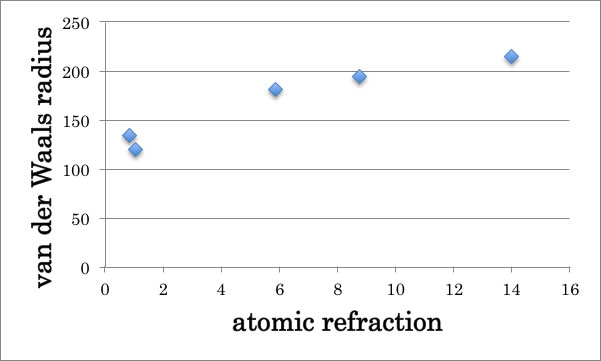So, it is believed that the speed of light in medium affected by VDW force.
Hydrogen and Halogen atoms are very simple, but Oxygen or Sulfur atom has much complicated state. For example, Oxygen has three type of atomic Refractivity in Lange’s handbook.

 Atom State Atomic Refractivity O -O-(H) 1.525 -O- 1.643 =O 2.211

For Nitrogen, there are more 20 type of state exist in Lange’s Handbook.
Even I admit atom state difference, I can’t agree to call X-membered ring effect as “Atomic Refractivity”.
And I don’t want call double bond as “Atomic Refractivity”.
For example, let’s think of 6 carbons molecule. I compare Benzene, cyclohexane, hexane, and Benzene’s refractive index is largest. We know aromatic compound’s refractive index is larger than alkane compounds.

 Hcode Name n MW Density RD 52 benzene 1.501 78.114 0.879 26.18 181 cyclohexane 1.4235 84.161 0.779 27.54 417 hexane 1.3723 86.177 0.659 29.74

But if I calculate RD with refractive index with density, the difference is very small and hexane’s RD is largest.
The hydrogen numbers are increasing 6, 10, 12, and Hydrogen’s effect for RD is increasing with 1.1(atomic refractivity)*Hydrogen#. As the result, they need assign 1.733 * double bond#.
But it is very strange to me. The double bond effect is already included into the density. When increase double bonds, the molecular shape are becoming more planner. Then the density becomes larger. What the atomic refractivity of “Double Bond”  do in the medium?  What the atomic refractivity of “X-member ring”, do in the medium?

So, I start to build my own QSPR model to predict refractive index.
I have the refractive Index data listed in Organic Solvents（4th Edition)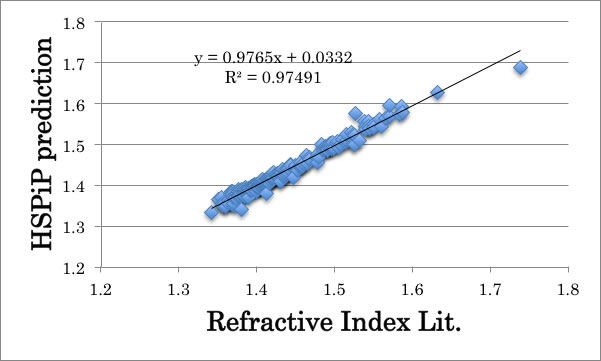I already built QSPR scheme to predict Refractive Index and that function is implemented into HSPiP. I determined 167 types of Functional Groups and get result like above.
So, we can’t expect higher square correlation factor (=0.97491) with atomic refractivity.

At first, I need to make table like below. I try to make RD estimating scheme with only number of atoms.

 Hcode (n^2-1)/(n^2+2)*Mvol C# H# Br# Cl# F# I# N# O# S# 303 24.18310878 2 6 0 0 0 0 0 1 1 1021 41.32190037 7 7 0 0 0 0 1 3 0 900 42.75388212 10 16 0 0 0 0 0 0 0 …. 604 36.82166188 8 8 0 0 0 0 0 0 0 851 35.16177053 8 7 0 0 0 0 1 0 0 867 53.32450722 12 22 0 0 0 0 0 0 0

Then calculate Multiple Regression program and get each coefficients.
(n^2-1)/(n^2+2)*Mvol= 3.7710*C＃+0.4106*H＃+8.2263*Br＃+5.4481*Cl＃+0.2692*F＃+13.5379*I#+3.1246*N#+1.7385*O#+8.3597*S#+1.06047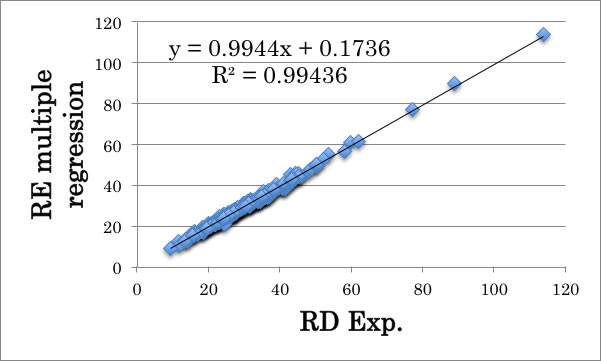The square correlation factor is 0.99436, so the accuracy of this scheme is very high.

If I plot these Multiple Regression coefficients to each atom’s VDW radius, I got very similar chart with Atomic Refractivity to VDW Radius.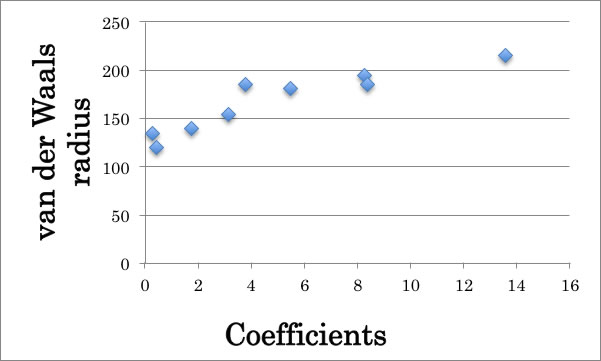Then from calculated RD and MVol, calculate Refractive index and compare them to experimental values.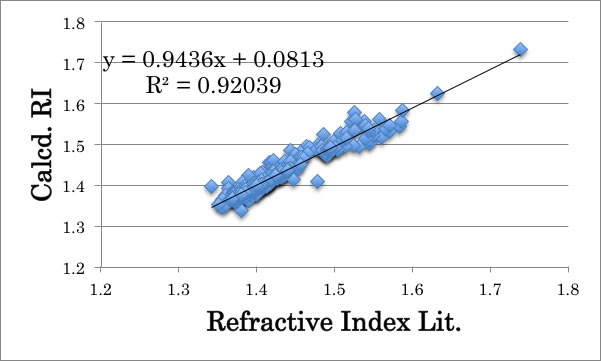The result is not good (R^2=0.92039).

When to get RD, I use calculated MVol, and when I calculate refractive Index I use same calculated MVol. So I need much much accurate estimation for RD.

Then the problem is which descriptors to use. I don’t want to use bond order nor X-membered ring.

The first thing to do is check which compounds are poor estimation.

 RI(lit.) - RI(Calc) Name 0.06795 dimethyl sulfoxide DMSO 0.04282 o-nitroanisole -0.03294 alpha-pinene 0.03624 1,2-Dimethoxybenzene 0.04044 styrene -0.03696 Phenyl Acetonitrile -0.02132 bicyclohexyl -0.05360 thiophene -0.02495 trans-decahydronaphthalene 0.03766 o-toluidine -0.04129 methyl cyanoacetate 0.03448 o-cresol 0.02007 2,2,3-trimethylpentane -0.01933 dibenzyl ether 0.03912 aniline -0.04449 chloroform -0.04351 propionitrile -0.05631 acetonitrile -0.02528 hexanenitrile -0.01929 octanenitrile

At first, DMSO is out of line.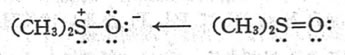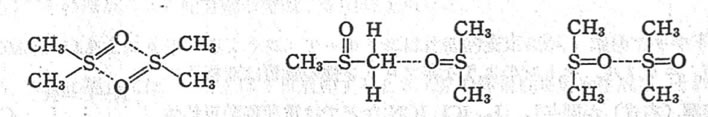DMSO makes very strange hydrogen bonding cluster like above at lower temperature, and density or Refractive Index of this compound is so unusual. So I delete DMSO for this research.
And the 6 Nitrile compounds are in the table. Every Calculated RI becomes large. So they introduce atomic refractivity of “triple bond”. I dislike the concept of atomic refractivity of XXX-bond, so I try to think other descriptors.

When we think of halogen atoms, we found that the VDW radius and atomic refractivity has correlation. If molecular interaction force affect to speed of light, I can build new scheme to predict RD.
Now I am working Hansen Solubility Parameters (HSP). And HSP divide heat of vaporization energy into 3(4) part.
dD: Dispersion energy (VDW energy)
dP: Polarity energy
dH: Hydrogen bonding energy (dH is divided Donor Acceptor. dHdo, dHac)
π-π stacking energy or other energies are put into dH.
Nitrile compounds have very large dP. So using HSP is reasnable.

And molecule’s shape also affects RD, so I add Ovality.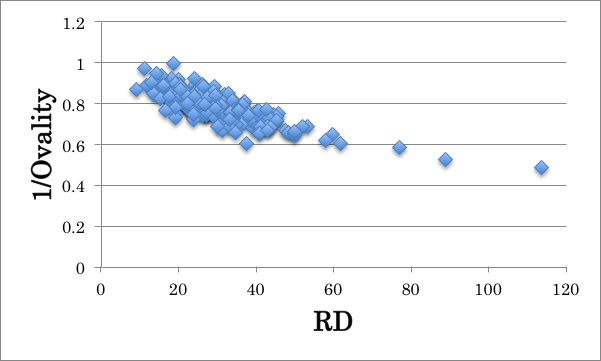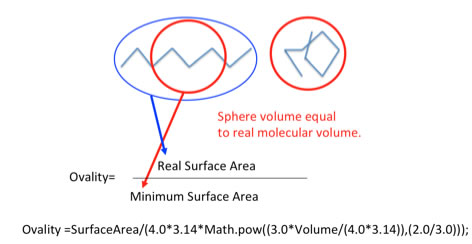At first, prepare the table

 Hcode RD C# H# Br# Cl# F# I# N# O# S# dD dP dHdo dHac 1/ovality 534 14.04 1 3 0 0 0 0 1 2 0 16.7 15.7 0.1 6 0.855 397 11.62 1 3 0 0 0 0 1 1 0 17.7 20.3 14.6 14 0.891 633 24.13 4 4 0 0 0 0 0 0 1 18.8 4.4 0.1 7.2 0.889 156 19.88 1 1 0 3 0 0 0 0 0 17.2 5 6.4 0.1 0.918 .... 1021 41.32 7 7 0 0 0 0 1 3 0 19.3 9.6 0.1 5.7 0.733 403 18.10 4 4 0 0 0 0 0 1 0 17.5 6.6 0.1 6.3 0.923 10 11.30 2 3 0 0 0 0 1 0 0 16.2 16.2 2.1 7 0.975 604 36.82 8 8 0 0 0 0 0 0 0 17.8 2.7 0.1 3.5 0.799 889 41.18 8 10 0 0 0 0 0 2 0 18.6 6.8 0.1 5.9 0.730 1041 22.84 4 5 0 0 0 0 1 2 0 16.9 13.7 1.2 9.5 0.779 488 18.60 1 3 0 0 0 1 0 0 0 18.1 6.5 0.1 5.2 1.000

Then build QSPR model.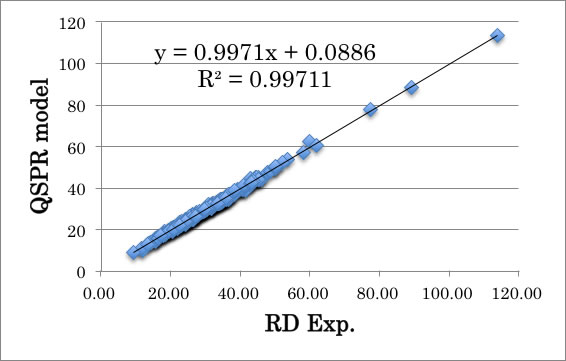Then convert RD to Refractive Index.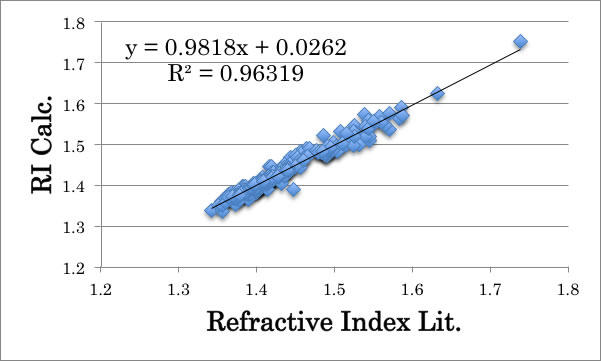When I introduced HSP and Ovality, RI prediction improve R^2=0.92039 to R^2=0.96319. It is still behind the Functional Groups (167) base calculation (R^2=0.97491), but this scheme use only 14 descriptors.
And I get knowledge of non-linear interaction between inputted descriptors.
For example, increasing RD interactions:
Hansen dD * N#  +1.2082
Hansen dP * S#  +1.2108
Decreasing interactions:
C# * N#  -0.8569
Hansen dHac * S# -0.6870
Then when I design high refractive index material for plastic lens, Sulfur atom increases RI, and Hansen dP is also high, sulfur effect will enhanced. But if Hansen dHac is also high, sulfur effect is canceled to half.
So to have complete QSPR scheme is very important to design optical materials.

I did not explain how to estimate Mol Volume here, but, it is also very important technology.

Anyway, Atoms in the medium determine Refractive Index roughly. And Molecular interaction parameter = HSP and ovality are very good descriptors to understand Refractive Index completely.

To estimate Surface Tension, Macleod-Sugden scheme is very popular.
γ^0.25*MW/(D-d) = P (parachor)
D: liquid density、ｄ: Vapor density

It is very well known fact that Molar refractivity [RD] and Parachor has very good correlation.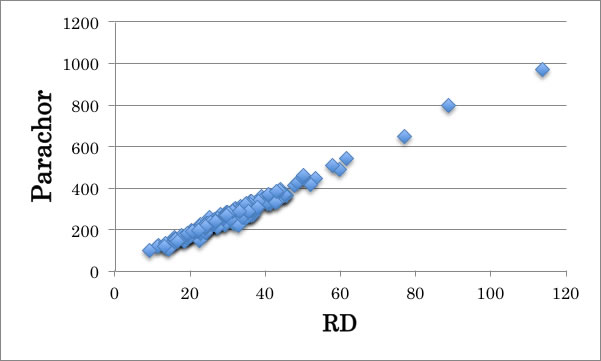So, if you know the Refractive Index and density, you can calculate Surface Tension.

The unit of RD is volume. And RD and Critical Volume (Vc) has also good correlation. This fact is also very popular.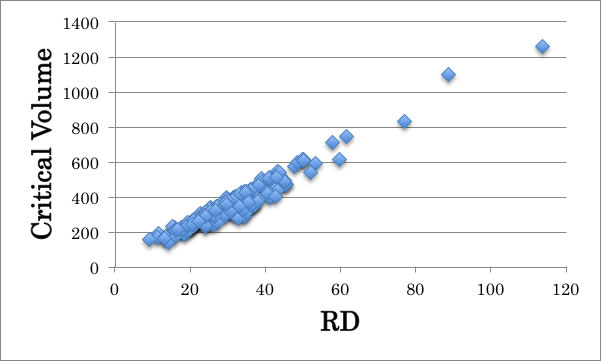So, RD is very useful for Corresponding state theory properties estimations such as Yen-Woods density estimation.

The data of temperature dependency of Refractive Index are so few. In the hadbook of Organic Solvents, I found only 120 compound's data. Molar refraction RD is thought to be temperature independent property and introduce temperature dependency term with density. I checked the correlation of dn/dT with other descriptors. I found highest correlation is Thermal Expansion m term. The Thermal Expansion Coefficient is calculated with next scheme.

TEC=a*(1-T/Tc)m

Tc: Critical temperature, a and m are compound dependent parameters.

when I plot thiophen's density to temperature, i will get one curve.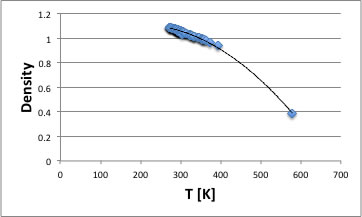The dDensity/dT is the TEC.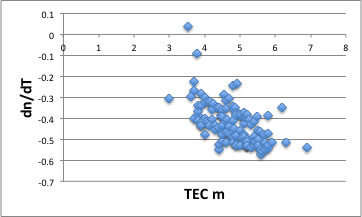And dn/dT has the largest correlation with TEC m.